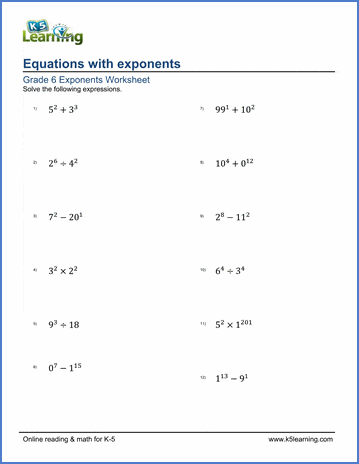## Exponent notation and expressions

Our grade 6 exponent worksheets expand our use of exponent notation and include whole number, fractional and decimal bases, negative exponents, and expressions and equations with exponents.

## Exponents

Whole numbers as base 73 =
Whole numbers as base (harder) 524 =
Whole numbers, fractions and decimals as base (0.2) =

## Negative or zero exponents

Negative or zero exponents (whole number bases) 3-1 =
Negative or zero exponents (0.8)-2 =

## Equations with exponents

Write expressions using exponents 0.6 x 0.6 x 0.6 =
Equations with exponents 24 -33 =
Equations with exponents, (negative bases) 33 - (-100)2 =
Equations with exponents, (fractional bases) 0.82  x 0.52=